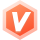2021-11-14 21:32

# 想知道这些题的正确答案

1题

#include <stdio.h>

int main()

{

char c='z';

printf("%c", c-25);

return 0;

}
2题

7
7.000000
2.000000
2

3题

4题

<=
=
%
&&

5题

6题

#include <stdio.h>

int main()
{

printf("*\n");

printf("**\n");

printf("***\n");

printf("****\n");

printf("*****\n");

return 0;
}

7题

#include <stdio.h>

void main()

{

int a, b, d = 241;

a=d/100%9;

b=(-1)&&(-1);

printf("%d,%d", a, b);

return 0;
}

8题

void main()
{
int m = 10, n = 20;
printf( ,m);
printf( ,n);
}

9题

#include <stdio.h>

int main()

{

int k = -3;

if (k <= 0)

printf("####")

else

printf("&&&&")

}

10题

1） 从键盘输入数据，调用scanf()函数
2） 用if..else if..else语句判断三个数的最大值

11题

#include <stdio.h>

int main()
{

int a = 10, b = 40, c = 20, t = a;

if (t < b)

{

``````            ;
``````

}

if ( )

{

t = c;

}

printf("%d %d %d中的最大数是 %d\n", a, b, c, t);

return 0;

}

• 写回答
• 好问题 提建议
• 追加酬金
• 关注问题
• 邀请回答

#### 3条回答默认 最新

•Roc-xb 后端领域优质创作者 2021-11-15 03:28
最佳回答

1、a
2、7.000000
3、运算符类型对应表达式的类型。
4、%
5、不对
6、

``````*
**
***
****
*****

``````

7、2,1
8、"%d\n" "%d\n"
9、不能编译通过，原因是printf语句后面缺少分号。
10、

``````#include <stdio.h>
#include <stdlib.h>

int main()
{
int a,b,c,max;
scanf("%d%d%d",&a,&b,&c);
if(a<b) {
max=b;
} else  {
max=a;
}
if(a<c) {
max=c;
} else {
max=a;
}
if(b<c) {
max=c;
} else {
max=c;
}
printf("%d\n",max);
return 0;
}
``````

11、

``````#include <stdio.h>
#include <stdlib.h>

int main() {
int a = 10, b = 40, c = 20, t = a;
if (t < b)
{
t=b;
}
if ( t<c)
{
t = c;

}
printf("%d %d %d中的最大数是 %d\n", a, b, c, t);
return 0;
}
``````
评论
解决 1 无用
打赏 举报 编辑记录